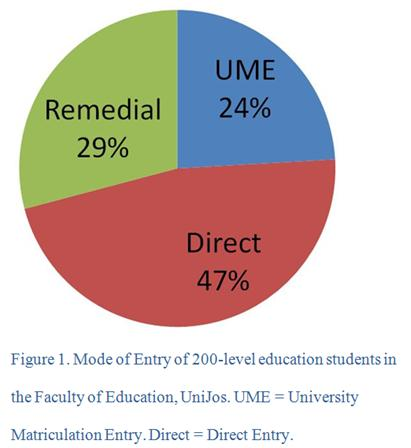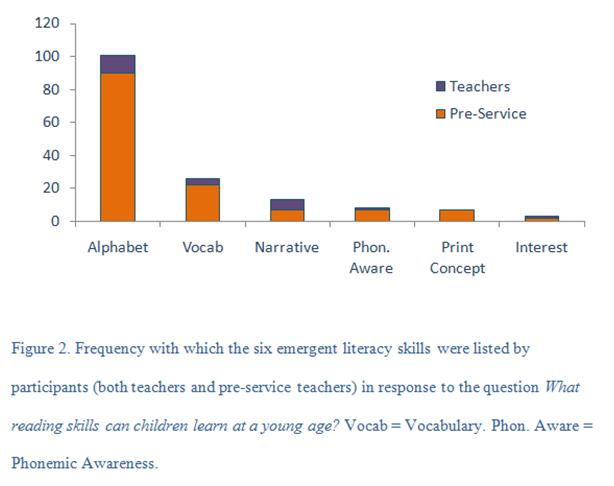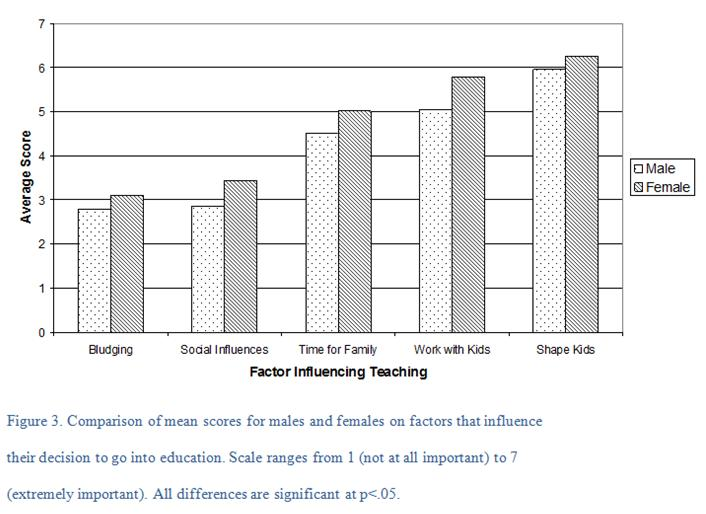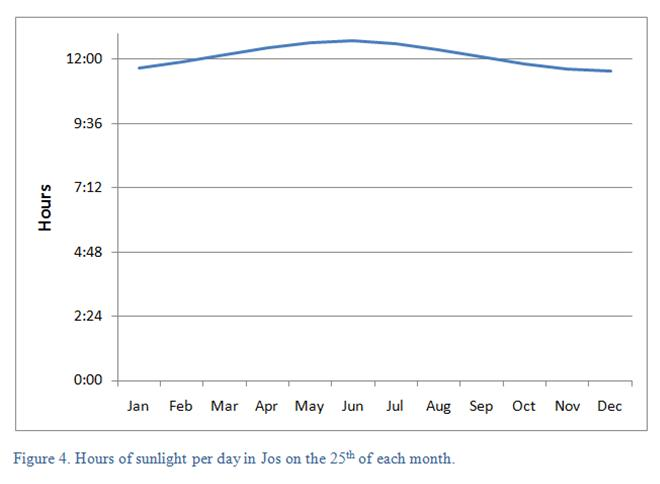Conducting Educational Research
Create Tables and Figures

Once the statistics have been calculated, the next step is to organize the statistics into tables and figures so the reader can easily read and interpret the statistics. The key to making both tables and figures is to make them clear, both in appearance and in interpretation. When you have finished a table or figure, critically look over it. Will the reader be able to understand what everything in the table/figure means? A good practice is to ask a peer to review the table and give their comments about how it can be more clear.

Tables

According to APA, tables enable a researcher to present a large amount of numerical data in a small amount of space. When developing a table, consider two seemingly contradictory requirements:

• Every table must be discussed in the text. This means that you must explain the key elements of a table in the body of your research report. When discussing tables, refer to them by the table numbers only, not where they appear in reference to the text. This is acceptable: "As can be seen in Table 3..." This is not acceptable: "The table above shows..." The rationale for this rule is that the placement of the table may change when the final document is produced.
• Readers should be able to interpret a table just by looking at the table, without reading the body of the research report itself. Therefore, each table should have a clear title that focuses on the key statistics within that table and all acronyms and abbreviations should be explained in the table notes.

Here are a few rules to keep in mind when creating a table:

• Table titles should be brief, but clearly explain the table.
• All similar entries in the table should carry the same number of decimal points. In other words, every entry within the same column should have the same number of decimal places. In general, whole numbers such as degrees of freedom should have 0 decimal points, p-values and correlations should be rounded to three decimal places (thousandths place value), and all other numbers (aka means, standard deviations, t-values, F-values) should carry two decimal places (hundredths place value).
• Tables typically have a horizontal rule at the top, bottom, and after the table labels. Vertical lines are rarely used according to APA regulations.
• If you are not the original author of the table, you must cite the source of the table in a note at the bottom.
• Once the tables have been assembled and placed in the text, number the tables starting from 1 in the order that they appear in the work.
• The decimal points must be lined up in each of the columns. This makes the table easier to read.

APA (2001, p. 175) gives this checklist when developing tables:

• Is the table necessary?
• Is the entire table, including the title, headings, and notes, double spaced?
• Are all comparable tables in the manuscript consistent in presentation?
• Is the title brief but explanatory?
• Does every column have a column heading?
• Are all abbreviations, special use of italics, parentheses, and dashes, and special symbols explained?
• Are all probability levels correctly identified, and are asterisks attached to the appropriate table entries? Is a probability level assigned the same number of asterisks in all tables in the manuscript?
• Are all vertical rules eliminated?
• Will the table fit across the width of the page?
• If all or part of a copyrighted table is reproduced, do the table notes give full credit to the copyright owner?
• Is the table referred to in the text?

Below is an example of a table that follows APA format: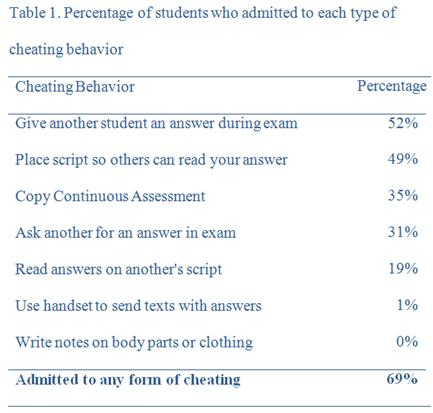Each of the tables below has some problems. Identify the problems in each of the tables.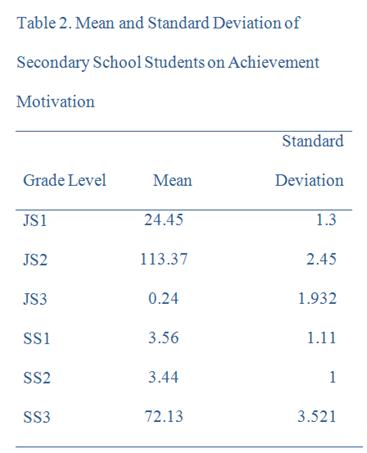Notice the numbers in this table. Under the Mean heading, all numbers have the same decimal places (two), but the decimal point is not lined up. It is not easy to see that JS2 has the highest number. Under the Standard Deviation header, the numbers are all rounded to different decimal places. All numbers in this column should have the same number of decimal places: two. Here is a corrected version of the table: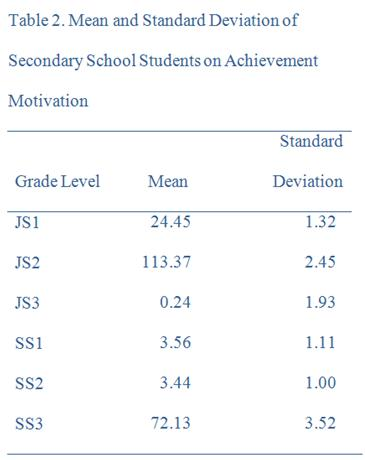What is wrong with this table?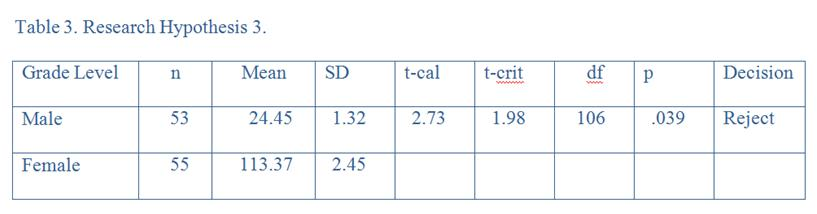First, the table has vertical rules. Second, the title of the table does not explain what the table represents. A more detailed title should be added. Below is a corrected version of the table. Note that this is not the "APA Format" for presenting the results of a t-test. The APA Manual does not give guidance on t-test tables. Indeed, it is often more common for t-test results to be written in the text instead of being presented in a table. For example, one might say "Females were found to have significantly more knowledge of child development than males (t(106) = 2.73, p<.05)." The degrees of freedom are placed in brackets immediately after the t. Then the t-calculated value is stated, followed by the signficance of the result (p<.05). However, in Nigeria, the table below is a typical method for reporting the results of a t-test.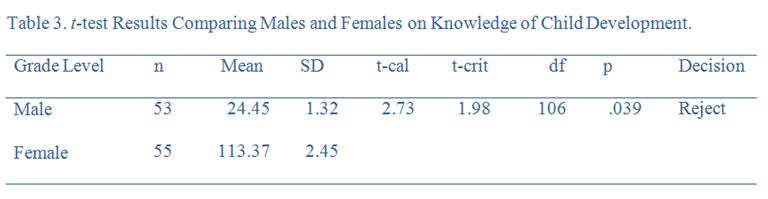What's wrong with this table?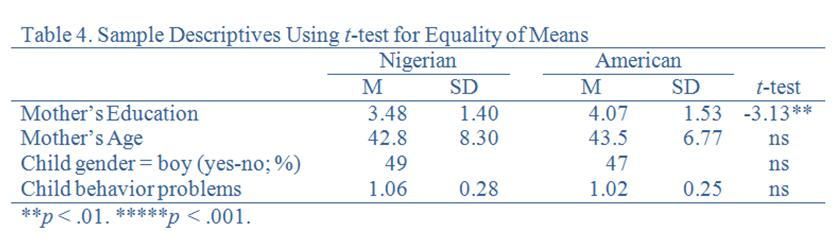Most simply, the table is not double-spaced. However, the major problems with this table are more complex. First, the table does not explain what M and SD stand for. It is standard that M is Mean and SD is Standard Deviation, but this should still be clarified in a note at the bottom. More importantly, the mean for Mother's Education is 3.48. What does this mean? That mothers only attended 3.48 years of school? This is highly unlikely. The author probably coded education - perhaps 1 was none, 2 was primary only, 3 was secondary, etc. However, the reader does not know this! A note must be added explaining the coding. Mother's age is understandable. However, the next line is also confusing: Child gender = boy (yes-no; %). Presumably, the first part means that 49% of the Nigerian sample was a boy, but what does (yes-no; %) mean? Likewise, what does 1.06 mean for child behavior problems? Is this high (perhaps the range was 0 to 2), or is this low (perhaps the range was 1 to 6)? Without this information, this table is meaningless. Below is a corrected table with a note at the bottom to explain the table.Figures

Figures can be an excellent way for readers to quickly understand and easily interpret the statistical findings. Graphs effectively illustrate means, frequencies, and percentages. Just like tables, figures should also be fully understandable without reading the text, but also be referenced in the text. Figures should also be numbered consecutively. Legends and notes should be included so the reader can easily interpret the figure. One difference is that figures do not have titles. Instead, a caption at the bottom of the figure functions both as a title and an explanation of the figure.

There are easy rules for determining the format and style of a graph. The researcher must use his/her expertise and judgment to determine whether to use a line graph, pie graph, bar graph, etc. and on developing the presentation of the graph. Again, it is helpful to have a peer review the chart to determine whether there might be a better way to assemble the graph.

Here is the APA (2001, p. 201) checklist for figures:

• Is the figure necessary?
• Is the figure simple, clean, and free of extra detail?
• Are the data plotted accurately?
• Is the grid scale correctly proportioned?
• Is the lettering large and dark enough to read? Is the lettering compatible in size with the rest of the figure?
• Are terms spelled correctly?
• Are all abbreviations and symbols explained in the figure legend or figure caption? Are the symbols, abbreviations, and terminology in the figure consistent with those in the figure caption? In other figures? In the text?
• Are the figures numbered consecutively with Arabic numerals?
• Are all figures mentioned in the text?
• Are figures that are being reproduced or adapted from another source given proper credit in the figure caption?

Below are some sample figures for percentage, frequency, means, and a line graph, respectively.